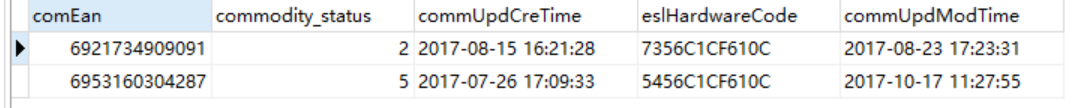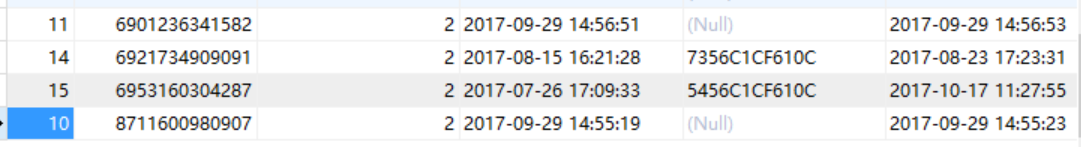1个回答

UPDATE table_a a
SET
name = (CASE id
WHEN
id
THEN
(SELECT
name
FROM
table_b
WHERE
id = a.id)
END),
nick_name = (CASE id
WHEN
id
THEN
(SELECT
other_nick_name
FROM
table_b
WHERE
id = a.id)
END)
where id in (select id from table_b);

``````这种方式能保证原来的数据在库中的顺序

``````